Function Repository Resource:

# DepthLeafCountSort

Sort expressions by depth and leaf count

Contributed by: Jan Mangaldan (original work by Stephen Wolfram)
 ResourceFunction["DepthLeafCountSort"][list] sort a List of expressions list by depth and leaf count.

## Details and Options

ResourceFunction["DepthLeafCountSort"] effectively sorts based on the global structure of expressions, while Sort sorts "lexicographically," looking only at the "early parts" of expressions.
This is the method of sorting used to order theorems in A New Kind of Science, page 817.

## Examples

### Basic Examples (2)

Sort a List of expressions by depth and leaf count:

 In:=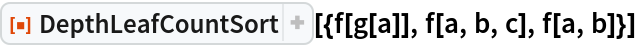Out=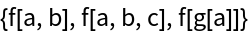Generate a List of expressions:

 In:=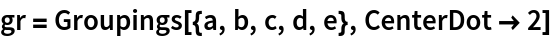Out=Show the tree forms of the expressions:

 In:=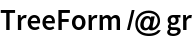Out=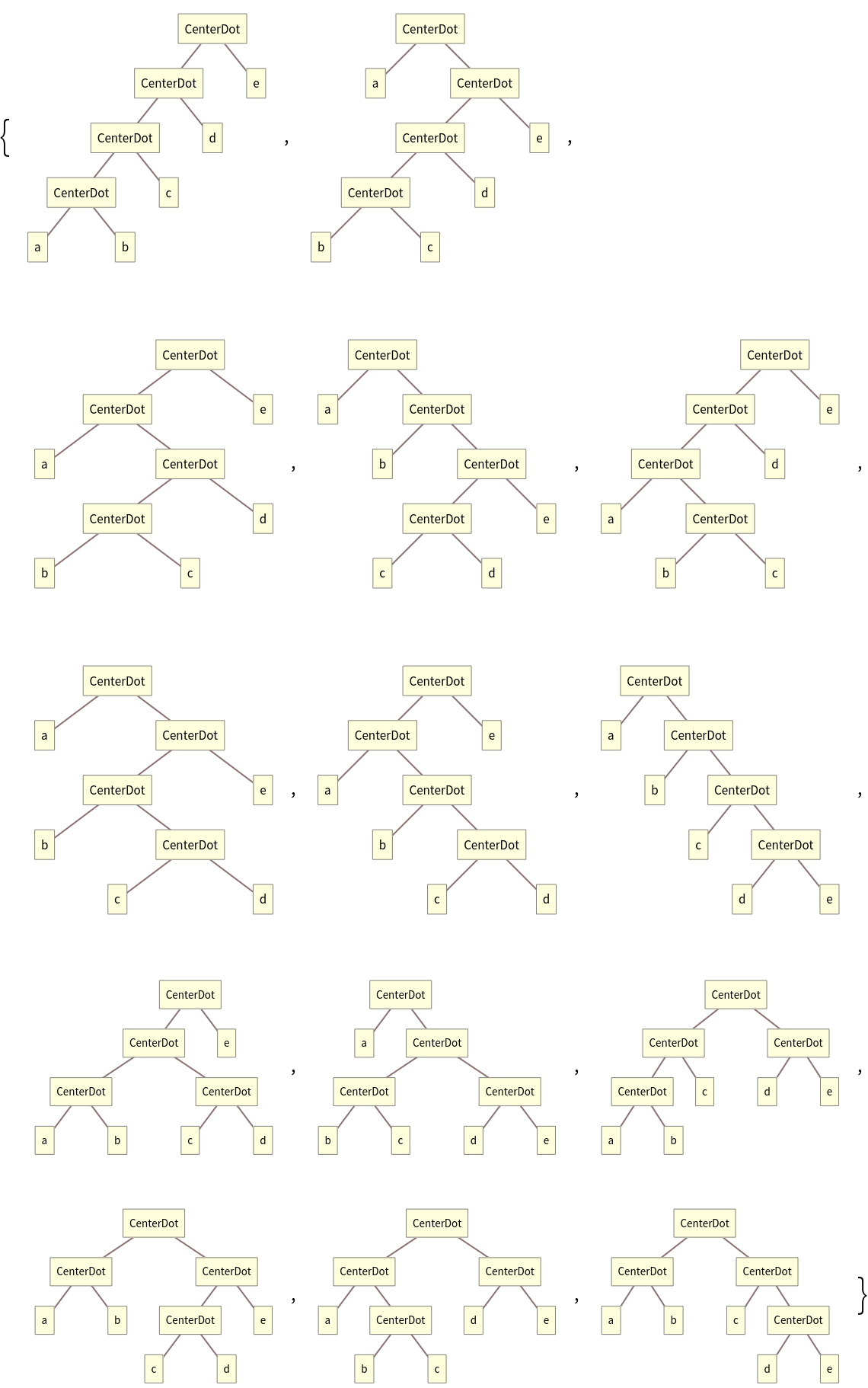Sort by depth and leaf count:

 In:=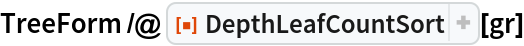Out=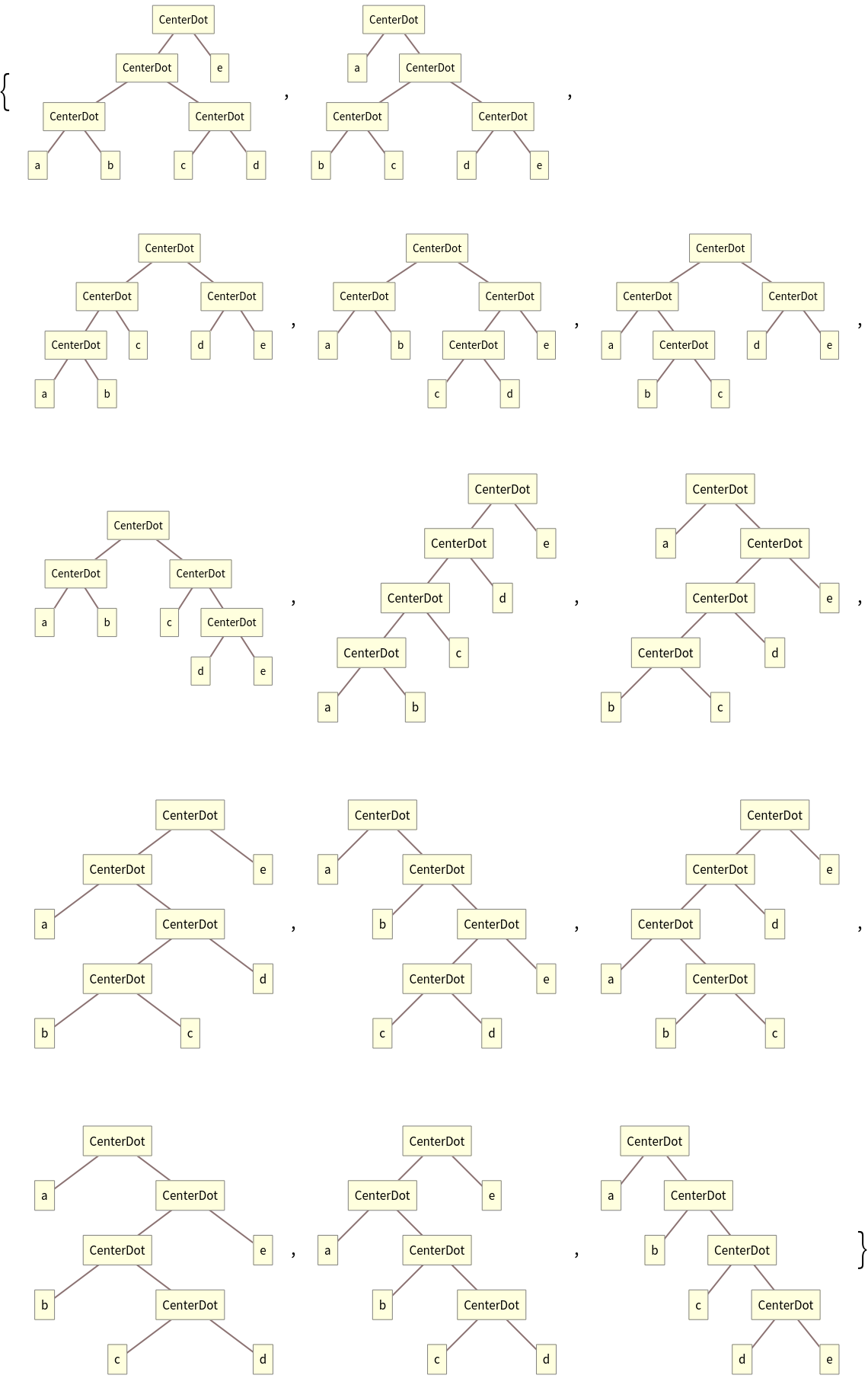### Properties and Relations (2)

Sort sorts first by length, then based on lexicographic ordering:

 In:=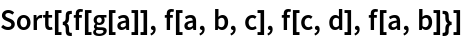Out=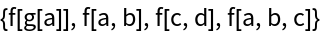DepthLeafCountSort sorts first by Depth then LeafCount:

 In:=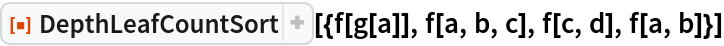Out=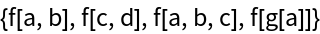Generate a List of expressions:

 In:=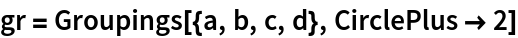Out=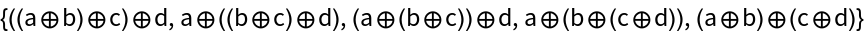Sort uses a lexicographic ordering:

 In:=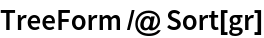Out=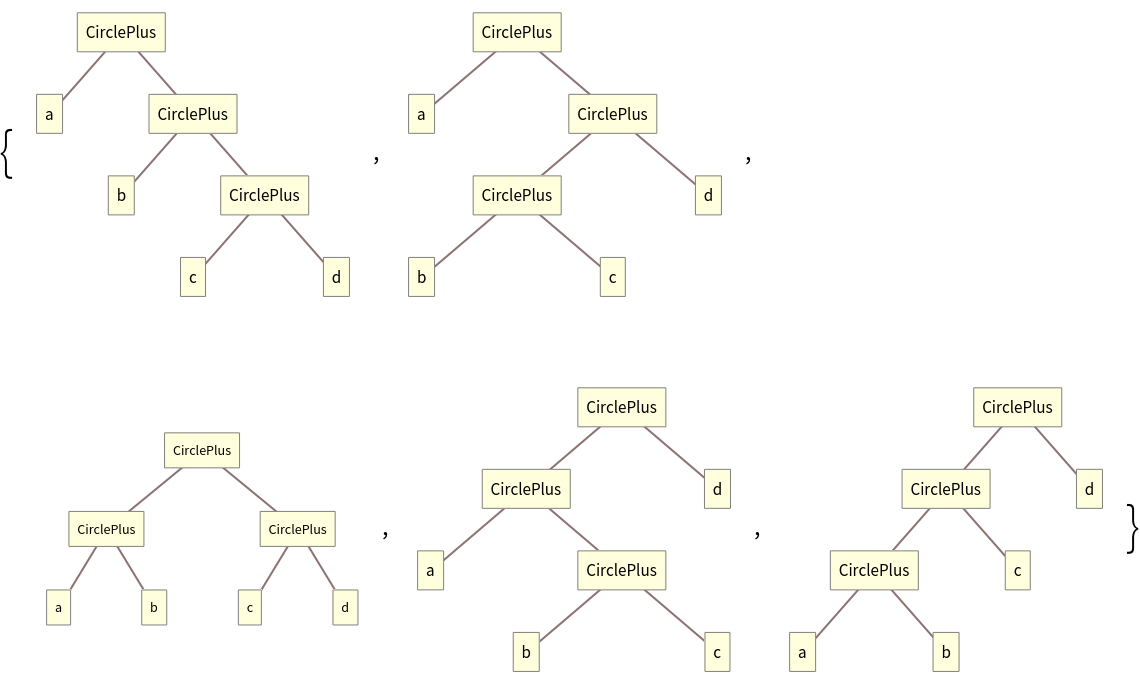DepthLeafCountSort orders expressions by their depth and then by their leaf count:

 In:=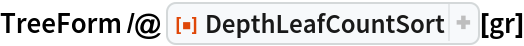Out=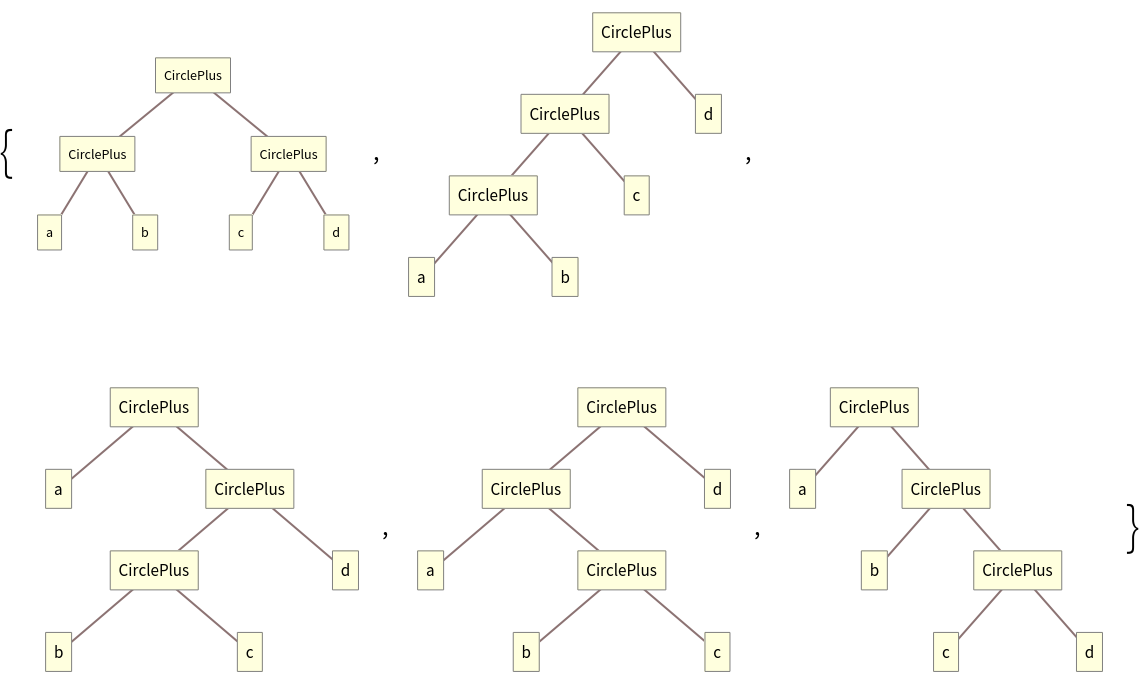An equivalent ordering using SortBy:

 In:=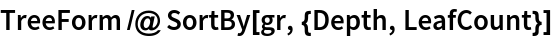Out=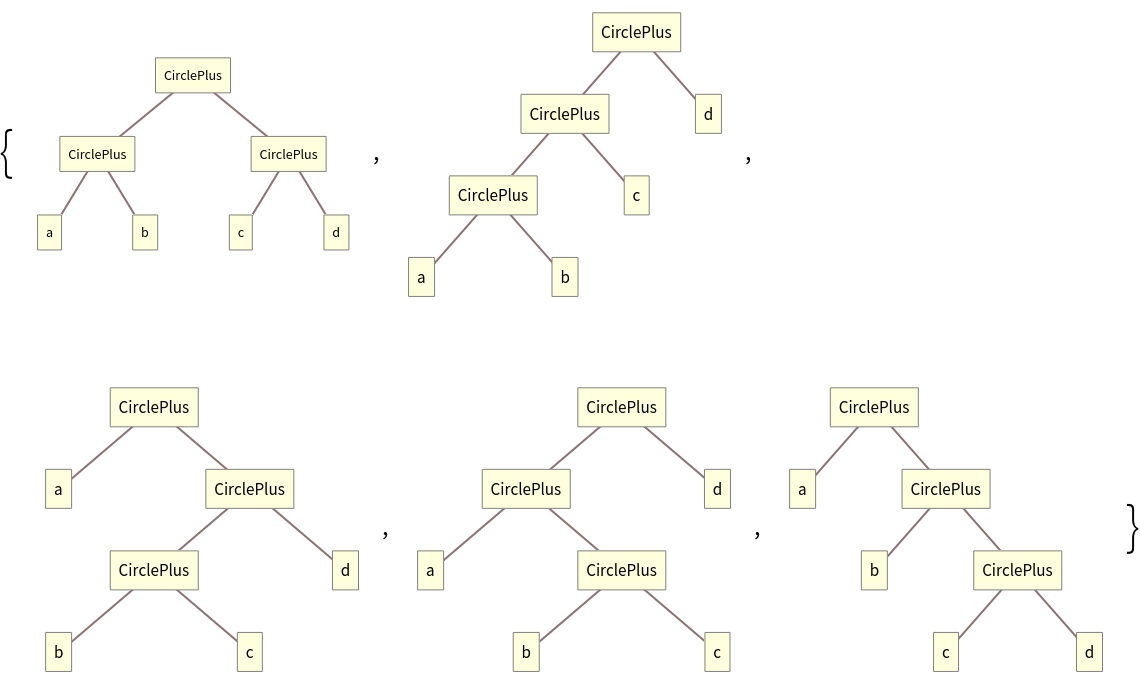## Version History

• 1.0.0 – 01 October 2020